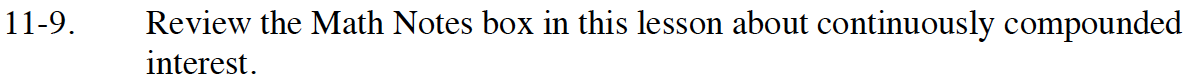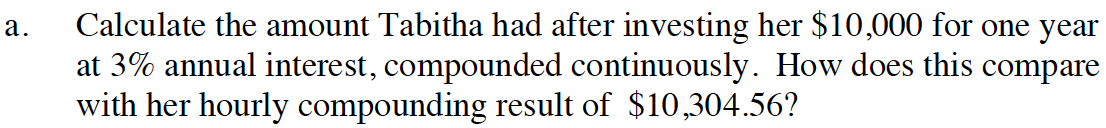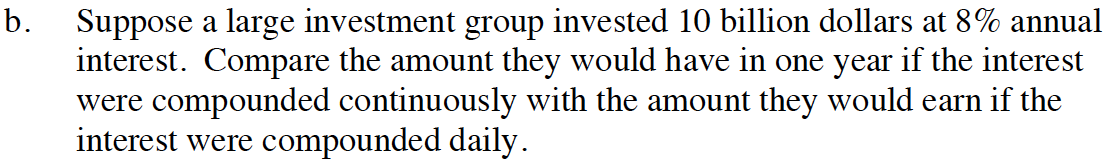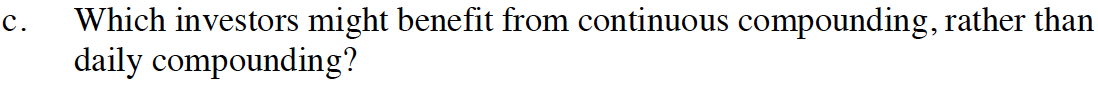Home > INT3 > Chapter 11 > Lesson 11.1.1 > Problem11-9

11-9.
1. Review the Math Notes box in this lesson about continuously compounded interest. Homework Help ✎

1. Calculate the amount Tabitha had after investing her $10,000 for one year at 3% annual interest, compounded continuously. How does this compare with her hourly compounding result of$10,304.56?

2. Suppose a large investment group invested 10 billion dollars at 8% annual interest. Compare the amount they would have in one year if the interest were compounded continuously with the amount they would earn if the interest were compounded daily.

3. Which investors might benefit from continuous compounding, rather than daily compounding?Remember to simplify within the parentheses before solving the exponent.$10,832,870, 680 and$10,832,775,720
Now find the difference.Is it better to have a larger starting amount or a smaller amount?